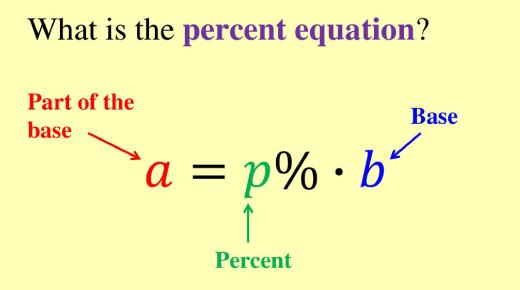# The Percent Equation: Test Your Math Skills! Trivia Questions Quiz

10 Questions | Attempts: 94
ShareSettingsThe quiz below is a percent equation designed to test your math skills! It is perfect for all the students that are having a hard time when it comes to solving some of the problems that have percentages or ratios. If you are in this category of students, be sure to give it a try and see how helpful it will be.

• 1.
A percent is...
• A.

A ratio that compares a number to 100

• B.

A comparison of two numbers by division

• C.

A ratio that compares a number to 1,000

• D.

A ratio that compares a number to 10

• 2.
A ratio is...
• A.

A ratio that compares a number to 100

• B.

A comparison of two numbers by multiplication

• C.

A comparison of two numbers by subtraction

• D.

A comparison of two numbers by division

• 3.
Write this ratio as a percent.  56 out of 100
• 4.
Write this percent as a ratio in word form. 38 percent
• 5.
Write this percent as a ratio in word form. 6%
• 6.
Where can you see percents?
• A.

Written as tests scores

• B.

When shopping for clothes at the mall

• C.

When leaving a tip at a restaurant

• D.

All of the above

• 7.
There are six colored marbles.  Two of the marbles are red, one of the marbles is blue and three of the marbles are green.  What percentage of the marbles are green?
• 8.
There are four colored pieces of paper.  One of the pieces of paper is pink, two of the pieces of paper are purple, and one piece of paper is orange.  What percentage of the pieces of paper is pink?
• 9.
Write this percent as a ratio in word form. 20 percent
• 10.
Write this percent as a ratio in word form. 60%

## Related TopicsBack to top
×

Wait!
Here's an interesting quiz for you.